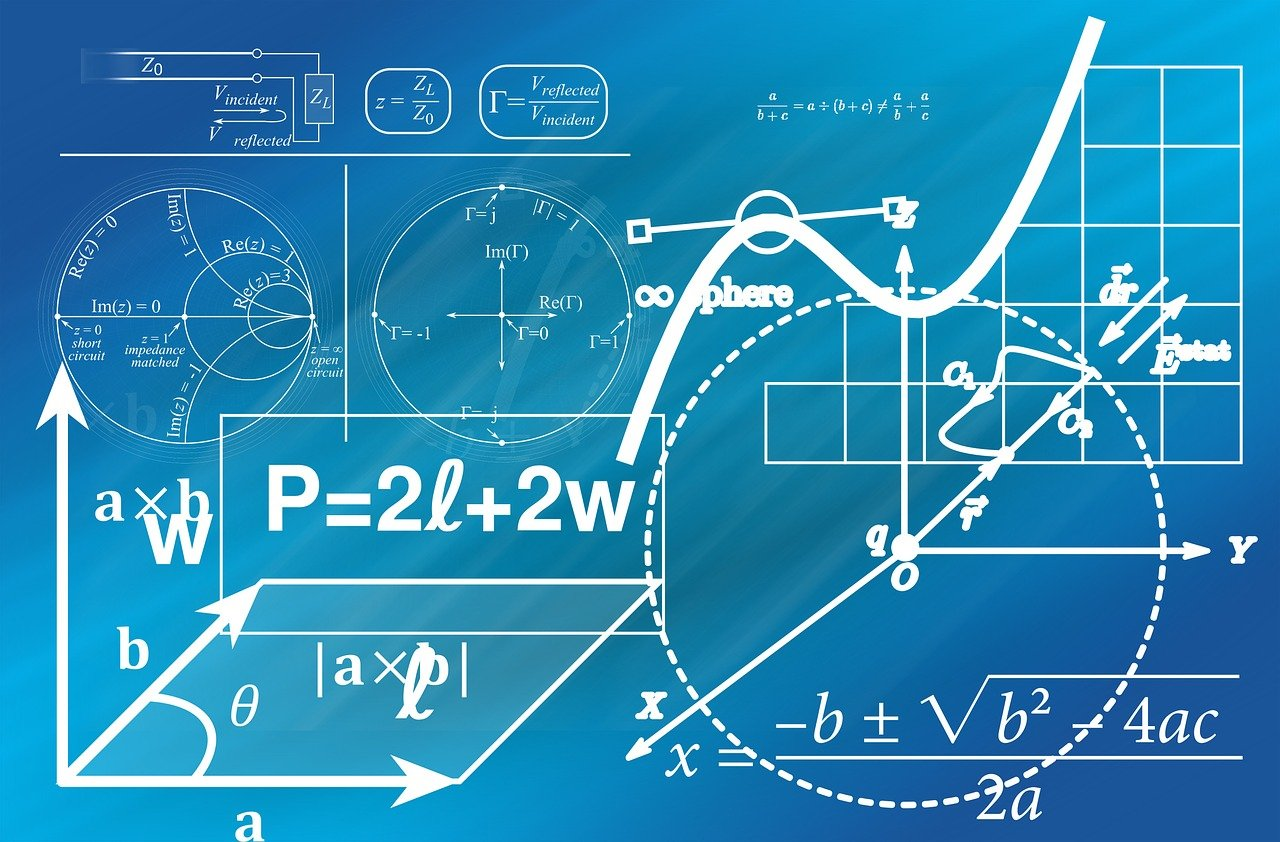# Arithmetical Calculations

Share this article!

Last Updated on November 7, 2018 by Katty

Don’t panic, I don’t want to teach you arithmetics! Instead, I’d like to help you learn how to talk about some of the easy arithmetical operations that apply in our daily life. Arithmetics is the oldest and simplest branch of mathematics, used by almost everyone. It is practiced daily by all for purposes as simple as counting objects to more advanced business and scientific calculations.

For your time in Italy, it would be helpful to learn how to do some simple operations in Italian. Such as:

• Quanto fa sei più uno? = How much/What is six plus one? 6+1 = 7Sei più uno fa/è uguale a sette = Six plus one is seven
• Quanto fa sette meno uno? = How much/What is seven minus one? 7-1 = 6Sette meno uno fa/ è uguale a sei = Seven minus one is six.
• Quanto fa cinque per quattro? = How much/What is five times four? 5×4 = 20

Cinque per quattro fa/ è uguale a venti = Five times four is twenty.

• Quanto fa sessanta diviso tre? = How much/What is sixty divided by three? 60:3 = 20

Sessanta diviso tre fa/è uguale a venti = Sixty divided by three is twenty.

• Sette diviso tre fa/è uguale a due con il resto di uno. 7:3=2

Seven divided by three is two and one over two (remainder one).

Fractions – Frazioni

In Italian fractions work as in English: the first number is a cardinal number, the second one is ordinal.

un mezzo ½ a half

un terzo 1/3 a third

tre quinti 3/5 three fifths

cinque mezzi 5/2 five over two

quattro mezzi 4/2 four halves

Some examples of how fractions are used in daily speech:

Ho già letto due terzi del libro = I have already read two thirds of the book.

Ne ha bevuto solo metà = He’s only drunk half of it.

In Italian when a fraction indicates a quantity the noun to which it refers is preceded by the preposition DI:

due quinti di olio = two fitfths of oil

un quarto di litro = one fourth of a litre

When the numbered object is feminine, ordinal numbers always have the masculine form:

un quarto di dolce = one fourth of cake

vorrei un quarto della torta = I would like one fourth of the cake

tre quinti di farina = three fifths of flour

tre quinti di questa farina = three fifths of this flour

Sometimes we say things like: “I would like a dozen roses (12 roses)” or “We’ll be about twenty people at dinner.” When discussing rough quantities in Italian here as some useful words and phrases:

• una decina = about ten
• una ventina = about twenty
• una trentina = about thirty
• una quarantina = about forty

Alla conferenza c’erano una ventina di uomini = At the conference there was about twenty men.

Quella donna è sulla trentina = That woman is about thirty years old.

Sarò lì tra una quarantina di minuti = I’ll be there in about forty minutes.

• un centinaio = about one hundred
• centinaia = hundreds
• un migliaio = about one thousand
• migliaia = thousands

Centinaia di feriti = hundreds of wounded

Un centinaio di persone = about a hundred people

Cento penne = one hundred pens

Un centinaio di penne = about one hundred pens

We can also express rough quantities with the word “paio” (pair):

Entro un paio di settimane saremo a Parigi = within a couple of weeks we will be in Paris

Vorrei darti un paio di consigli = I would like to give you a couple of tips

Ho comprato due paia di scarpe = I bought two pairs of shoes

Cinque paia di pantaloni = five pairs of trousers

Ratios / Proporzioni

a sta a b come c sta a x a:b = c:x a is to b as c is to x

Raising to a Power / Potenze

due alla seconda (potenza) / due al quadrato = two to the power of two / two squared

due alla terza / due al cubo = two to the power of three / two cubed

elevare alla quinta = to raise to the power of five

elevare all’ennesima potenza = to raise x to the power of n

Decimal Fractions / Decimali

tre virgola dieci = 3,10 = 3.10 = three point ten

zero virgola zero cinque = 0,05 = 0.05 = zero point zero five

zero virgola zero uno = 0,01 = 0.01 = zero point zero one

due virgola tre periodico = 2,3333…. = two point three recurring

Percentages / Percentuali

A percentage is a way to express a number as a fraction of a whole. For example:

dieci per cento = 10% = ten per cent

cinque virgola cinque per cento = 5,5% = 5.5% = five point five per cent

Il 20% dei lavoratori ha più di 40 anni = Twenty percent of the workers are over 40.

L’offerta è aumentata del 10% = The offer has risen by 10%.

Comments are closed.